# But what is a quantum phase factor?

## An intuitive approach from absolute scratch

7 hours. That’s how long it took me to understand what phase really is. I’m writing this so that you can achieve the same level of understanding in 11 mins.

So what is it?

Sit tight, and I’ll cover everything, I promise. The struggle you might have faced at first was that it appears to come out of nowhere, and seems to have no use. And most of whom that encounter it, accept its use case and move on, without truly asking why. This is the plug and chug approach.

I’ll be honest, not a fan of the ol’ plug and chug so I’m going to structure this so that it actually makes sense. For your sake, I’ll avoid the heavy quantum jargon unless it’s essential. We’ll start with a review of the basics, feel free to skip around if you’re already familiar with the state space.

# Laying the Foundation

Can you think of something truly random?

The flip of a coin?

Nope.

A number generator on a computer?

Nope.

Elon Musk’s tweets?

Almost, but no. If you want to appreciate true randomness, we’ll have to go down to the quantum scale, but first, what is true randomness? You might be thinking, “isn’t the flip of a coin random because it’s 50–50?”

Two points.

One, the probability distribution isn’t a determinant of randomness. Take for example the coin, which could be truly random yet have an 80–20 chance of being heads or tails.

The second point being, true randomness is the complete inability to predict the outcome, even if you have access to an infinite set of data. Your coin flip isn’t truly random, because if we knew everything about the coin being flipped and everything about the air around it, we’ll be able to predict the outcome. This is not the case when we’re talking about subatomic particles and radioactivity, it is fundamentally random.

Physicists needed a way to describe the state of such a random particle. And what did they come up with? Well — as obvious as it may sound — they came up with a function, namely the wave function, to describe the probabilities of the particle being in one state or the other.

So this is what superposition is — the probability distribution for a quantum particle. That’s it.

## On a geometric representation

If we have an electron that could be in two binary states, let’s say black and white for simplicity, the system is said to be in superposition if there are multiple possible states (black or white) that it can be in, black or white. But we just don’t know until we measure the system because it’s truly random. We can express this geometrically as a line between the two possible basis states.The amplitudes are the coefficients in front of each state in superposition and are proportional to the probabilities of the electron being in each of those basis states, we’ll review this soon.

This notation with |and is called the ket notation, and things like|0⟩ are called kets. But don’t be thrown off by the unfamiliar terminology — a ket is just a vector. In this case, you can think of each ket as two binary states, 0 and 1 corresponding to black and white.

We know that all probabilities must add up to one, so that means that the length of that vector must be one. Let’s create a circle to better represent the possible vectors given the unit length, we’ll develop this geometric representation as we progress.

The vector is in the middle of the 1 and 0 state, telling us that it’s in superposition (probabilistic). So what does the 0.6 and 0.8 mean?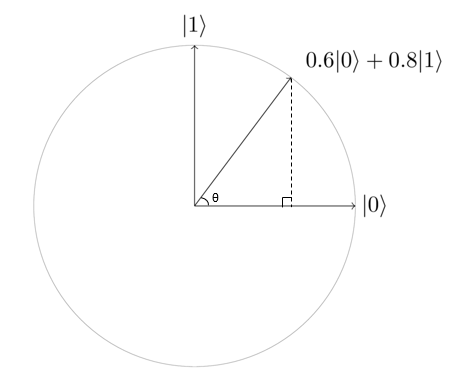cos(θ) = horizontal component ; sin(θ) = vertical component

The amplitudes of each basis state tell us the length of the horizontal and vertical components. In this case, the horizontal component (amplitude) is 0.6 and the vertical component (amplitude) is 0.8. The square of each will give us the probability of the superposition collapsing into a certain basis state.

Probability = |α|² or |ß|²

In this example, the probability of the state collapsing into |0> and |1> is 36% and 64%, respectively.

With the Pythagorean theorem, we know that the sum of the squares of each side equals the radius. Since the radius equals 1 — following the fact that the sum of probabilities cannot exceed 1 — we can sum up our findings and state this truth for any two amplitudes, denoted by Greek letters alpha and beta.

# Phases in Layman’s Terms

Up till now, I haven’t been forthright. You might have noticed that up to this point, the amplitudes we’ve been dealing with have all been real numbers. The reality however is complex — pun intended — let me explain!

The intention of this article is not to give you a math lecture, so I would recommend brushing up on your complex numbers if you don’t understand what that is.

The geometric representation (the circles) we’ve been using to describe the quantum state has been a real two-dimensional plane. In truth, the quantum state of a qubit is represented by a vector of length 1 (like we concluded with pythag) in a two-dimensional complex vector space known as state space.

Given that each axis is complex, that would imply that our coefficients alpha and beta are also complex amplitudes. I wish I could show you a picture of a two-dimensional complex plane, but that would entail four real dimensions, which of course we can’t visualize.

## What’s the point of the amplitudes being complex?

A perfectly reasonable question to ask. To answer that let’s think of our prior example, where we had two real amplitudes which constituted two real dimensions. When using real amplitudes, we’re constrained to one dimension for each amplitude, what do I mean by that?

Think of a number line. If you want to express the probability of (1/2) then your real amplitude for the given basis state would be (1/√2). That’s it. All possibilities for amplitudes lie on the number line, and there’s only one real amplitude for any magnitude.

Introducing complex numbers to the mix allows us an extra degree of freedom. Through the rotation of the complex vector, you can encode the same magnitude (1/√2) with an infinite number of configurations around a circle. The direction of these vectors is what we refer to as a phase factor. Phase sets the groundwork for quantum computing since it legitimizes interference effects, let’s explore this further.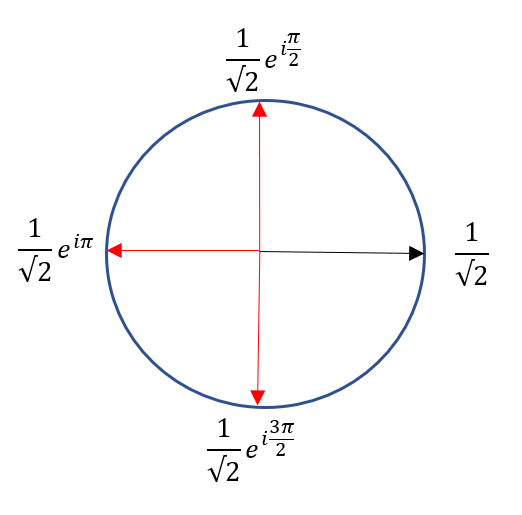One complex amplitude → Black: the only possibility given a real amplitude; Red: examples of unlocked possibilities with a complex dimension

An aside on exponential form for complex numbers: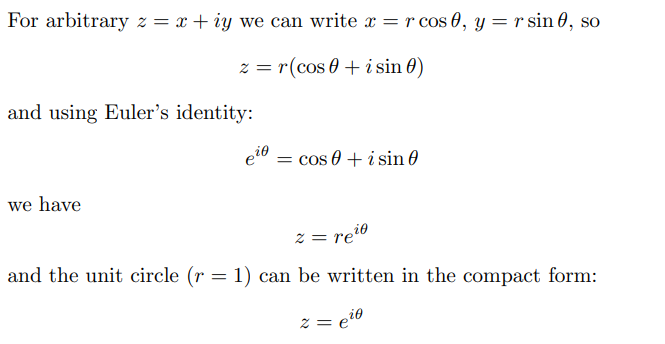Exponential form for complex numbers. Where theta is in radians

## But phases are only for waves right?

Yes but no. I’ll explain. Drawing connections from your high school trigonometry classes, you probably remember being told that a horizontal translation of a certain wave causes a phase shift.

Quantum particles display wave-particle duality. This came as a big surprise that matter sometimes also behaves like a wave in addition to its particle behaviour. So long story short, qubits — when in superposition — behave as a “matter wave” which opens the door for phase.

## What’s the point of phase?

Interference! The presence of phase implies that quantum particles exhibit interference effects, just like sound! Because the matter-wave can spread in space, it can destructively and constructively interfere at certain positions. Interference effects give rise to quantum advantage, as explained so eloquently in Quantum Computation (Scholarpedia):

A fundamental idea in quantum computing is to control the probability a system of qubits collapses into particular measurement states. Quantum interference, a byproduct of superposition, is what allows us to bias the measurement of a qubit toward a desired state or set of states.

As I said before, the same probability can be expressed in an infinite number of rotations as a phase factor. So realistically, there’ll be no difference if you measure two qubits with opposite phases again and again. They exhibit the same probabilities. That’s why the phase factor isn’t a directly observable quantity. On the other hand, the probability (|α|² or |ß|²) is an observable quantity since we can observe the qubit collapse into different basis states per that probability distribution.

But again, phases are useless when dealing with a single qubit, the power comes from interference effects inherited from the presence of multiple qubits. So yes, it may not be a tangible property. At least not with one qubit. But it certainly manifests itself through the interaction of qubits, and exploiting this property of interference is what lays the basis for the quantum advantage.

# Creating another geometric representation

Two dimensions won’t cut it. If we want to represent phases for each complex amplitude, we’ll need 4 dimensions. Hmmm, let’s see if we can get around that though. The path we’ll take to explain phase interference (waves) will stray from the convention, but I’d argue that it’s much more intuitive.

## A recap on interference

Recall that the only reason phase is important is because it brings about interference effects. And interference effects are only dependent on the difference in phase between the two waves (we will abstract basis states to waves for now). Therefore, we can say that it’s the difference that counts, and not the absolute value. For example, if the phase difference is π radians then the waves would cancel each other out.

We can conclude that the absolute value of the phase shift for both waves is meaningless to the interference. For as long as they both retain the phase difference, then the interference effect will be constant, and that’s what matters!

## Why the global phase doesn’t matter

As observed above, what matters is the phase difference. So think about it, if you’re describing the phase of two waves, it’s redundant to state both phases. The better approach is to just state the phase difference.

Guess what? You already know why the global phase doesn’t matter. Let’s attach some terminology to formalize our understanding.

The global phase is the absolute value of the phase shift for both waves. For example, wave one and two each have a phase of (π/2) radians and (3π/2) radians, respectively. In this case, the phase (π/2) is a global phase since the phase difference (what actually counts) is π radians → (3π/2 - π/2 ). Using the same example, we can define the relative phase (also known as the local phase) as the phase difference (π rad).

## Connecting it all back into quantum states

We’ve taken a circuitous — but rewarding — route to truly understand the intuition behind global and relative phases. Let’s connect it back to our geometric representation of quantum states.

Everything I said about phases, interference, global phase, relative phase, it all applies when discussing our quantum state as well! The only difference is that we’ve found a method to bypass the need for a fourth dimension by factoring out the global phase and replacing the second phase with the relative phase. We can represent this mathematically.

## Let the mathematics do the talking

So we know that a general qubit state can be written

with complex numbers, α and β, and the normalization constraint require that:

As previously stated, we can express the amplitudes in polar coordinates as: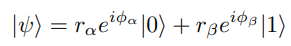General equation for a qubit state

with four real parameters:

However, the only measurable quantities are the probabilities |α|² and |β|², so multiplying the state by an arbitrary factor e^iγ (global phase) has no observable consequences, because: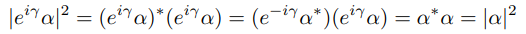How global phase vanishes when calculating probability

Therefore, we can factor out e^iΦ from the general equation:

Now, if you calculate the amplitude |ψ|², the factor (e^iΦ_α) in front will vanish by the argument above. This is why we called it the global phase. However, the relative phase is the phase difference noted as (Φ_α - Φ_β). This is an observable-ish quantity which manifests through interference effects.

Let’s consolidate the above equation into:

where r_α, r_β and Φ all real parameters.

Notice that this equation can be represented in 3-D, as the global phase is gone.

# An aside on the Bloch sphere

I know, I know, I’m really pushing it with this article, but I can’t resist myself not to share the Bloch sphere with you after showing you that a qubit state can be represented in three dimensions. I promise it’ll be quick.cos(θ) = horizontal component ; sin(θ) = vertical component

Remember this from the beginning? Well, it models a 2-D quantum state but since we need to incorporate the relative phase, we’ll need a third dimension. Also, let’s move the basis vectors to the poles of the sphere so that it looks better, this is the actual reason why the basis vectors are on the poles!

## Voila!A three-dimensional representation of a given quantum state where Φ is the phase factor and sin(θ/2) is the amplitude for the |0> state and cos(θ/2) is the amplitude for the |1> state

Why is theta divided by two?

Recall that all probabilities amplitudes can be shown when theta is :

0 ≤ θ ≤ π/2.

Our prior representation demonstrated this since the basis vectors were π/2 radians apart. However, now our basis vectors are at the poles of the Bloch sphere π radians apart. Since we doubled the angle between our basis vectors to get to the Bloch sphere, we need to divide an arbitrary angle on the Bloch sphere by 2 to go backwards and calculate the vertical/horizontal components.

For example, in our prior “Bloch circle” with the amplitude of 0.6, θ was 0.93 radians. This means that when converting the amplitude to the Bloch sphere, we’ll need to double it by two since the conditions for theta has stretched to:

0 ≤ θ ≤ π

In that respect, when measuring θ’ from the Bloch circle, we need to half it since it still works the same way as the old representation.

## General equation for a qubit state on a Bloch sphere

Therefore, an arbitrary single-qubit state can be written:

where θ and Φ are real numbers. The numbers 0 ≤ θ ≤ π, and 0 ≤ Φ ≤ 2π define a point on this unit three-dimensional sphere — don’t forget the radius is always one, at least for pure qubit states, but that’s an article for another day!

Take a moment to appreciate all of the prerequisite knowledge you gained to be able to truly understand the meaning behind the Bloch Sphere. People flaunt the Bloch sphere around but struggle to answer fundamental questions like why it’s three-dimensional; no further do you fall in that category.

# All you need to know

• An arbitrary state space is a two-dimensional complex vector space, which is then four real dimensions. The fourth dimension can be factored out as a global phase, so then what remains is a three-dimensional representation which is the Bloch sphere*.

*You could further reduce this to two real values under the normalization condition but we don’t want to get rid of relative phase in our visualization of a quantum state since it’s instrumental for interference.

If you enjoyed the article or learned something new, feel free to contact me on LinkedIn, or see what I’m up to in my monthly newsletter.

--

--